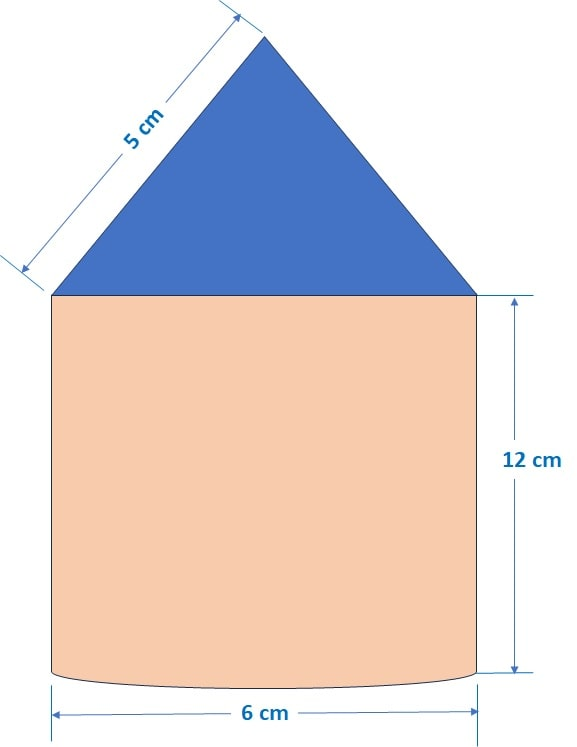Q) A rocket is in the form of a right circular cylinder closed at the lower end and surmounted by a cone with the same radius as that of cylinder. The diameter and height of cylinder are 6 cm and 12 cm, respectively. If the slant height of the conical portion is 5 cm, then find the total surface area and volume of rocket. (Use π = 3.14)

Ans:We are given that, diameter of cylinder, D = 6 cm ∴ radius of cylinder, R = 3 cm

∴  diameter of cone, d = 6 cm ∴ radius of cone, r = 3 cm

Height of the cylinder, H = 12 cm

Slant height of the cone, l = 5 cm

Step 1: Height of the cone, h =(slant ~ height^2 – radius ~ of ~ cone^2)

== 4 cm

Step 2:

Curved surface area of the cone =r l =(3) (5) = 15Curved surface area of the Cylinder = 2R H = 2(3)(12) = 72Base area of the Cylinder =R^2 =(3)^2 = 9∴ Total Surface Area of the rocket = Surface Area of Cone + Surface Area of Cylinder + Base Area of Cylinder

= 15+ 72+ 9= 96= 96 x 3.14 = 301.44 cm2

Therefore, the Surface Area of the Rocket is 301.44 cm2

Note: Here, base area of cylinder is taken because it is given that the cylinder is closed.

Step 3:

Volume of the Cone =r2 h =(3)2 (4) = 12Volume of the Cylinder =R2 H =(3)2 (12) = 108Volume of the rocket = Volume of Cone + Volume of Cylinder

= 12+ 108= 120= 120 x 3.14 = 376.8 cm3

Therefore, the Volume of the Rocket is 376.8 cm3

Scroll to Top